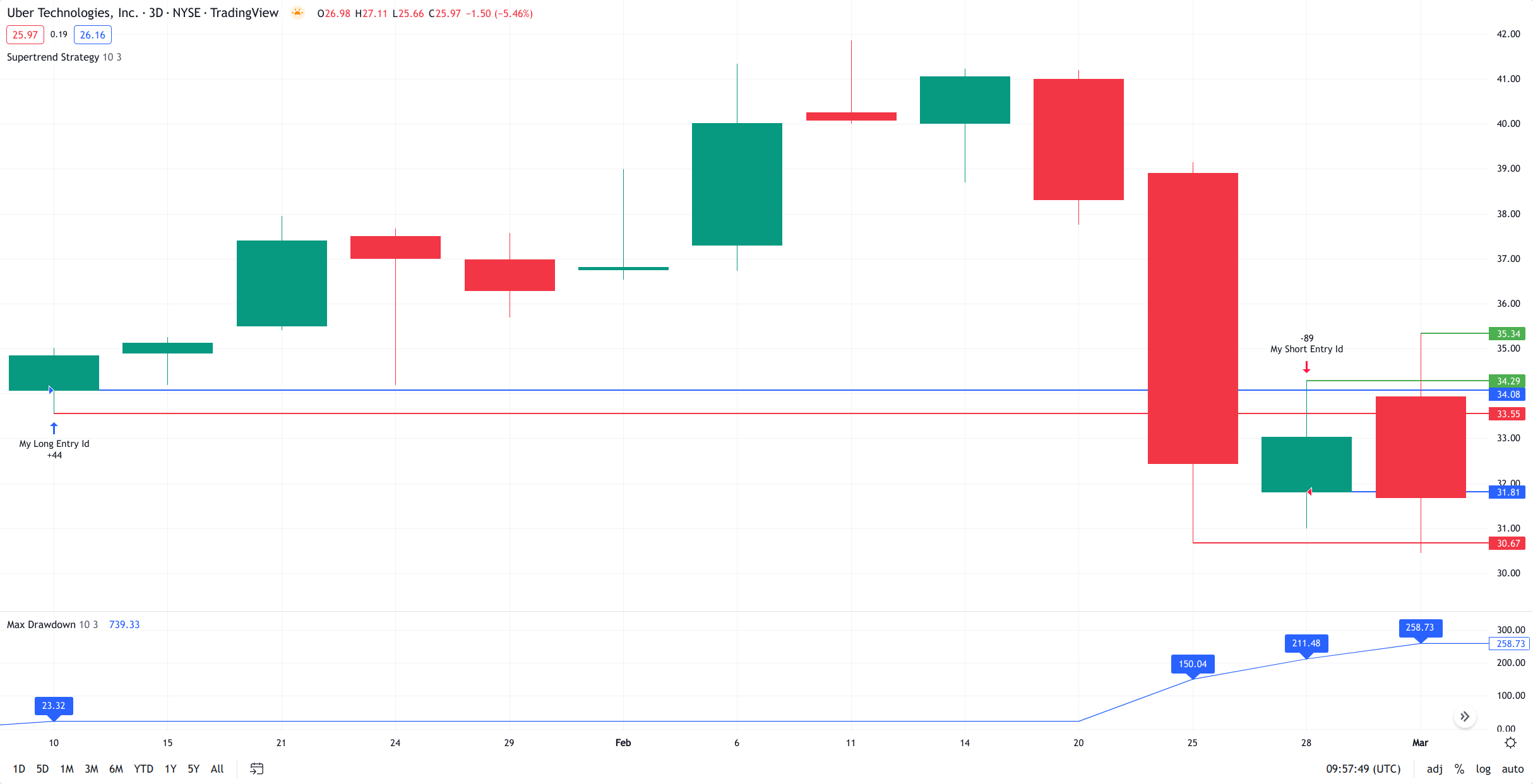# Performance Summary: Max Drawdown

Displays the largest drawdown of losses, i.e., the maximum possible loss that the strategy could have incurred among all of the trades it has made. This value is calculated separately for every bar that the strategy spends with an open position. To calculate the maximum drawdown, which is displayed on the Performance Summary tab in the Strategy Tester, we:

1. For each separate trade, calculate the Equity before the current trade’s opening. For the first trade, this value will be equal to Initial Capital.

2. For each trade, calculate Max Equity the strategy had before that trade opened. To do so, we take the strategy's Initial Capital and all of the Equity values from the trades that were already closed by that moment and find the largest number among these values.

3. Calculate the drawdown of the strategy for each bar where the strategy was in a market position. For long trades, it is calculated by the following formula:

Max_Equity - Equity_on_Entry + Numbers_of_Contracts * (Entry_Price - Current_Low)

For short trades, the formula will look like this:

Max_Equity - Equity_on_Entry + Numbers_of_Contracts * (Current_High - Entry_Price)

Note that if you calculate the drawdown for the closing bar of a trade, you should also account for the intrabar price movement, which goes from Open to High or Low (whichever is closest), then to the other value of that pair, and then to Close. So if the trade was closed on the bar's open, Open will be considered both the maximum and the minimum value of that bar.

4. After finding the drawdown for the current bar, find the maximum value among all of the drawdown values we have calculated. This will be the Max Drawdown for the current position of the strategy.

Let's look at how Max Drawdown is calculated at this example:We use the Supertrend Strategy with Initial Capital equal to 10000 USD and open NYSE:UBER on the 3D timeframe as the symbol.

We are looking at the first trade, so our Max Equity and Equity on Entry will be equal to the Initial Capital. On first trade opened on Jan 10, 2020, the strategy entered long and bought 44 contracts for 34.08 = 1499.52 USD worth of stock.

At the same bar the price reached a minimum of 33.55. If we sell our stocks at this point, our drawdown would be 10000 - 10000 + 44 * (34.08 - 33.55) = 23.32. This is the only drawdown value we have, so for the time being it is also the Max Drawdown.

At the bar for Feb 25, 2020, the price reached a minimum of 30.67. The drawdown on this bar will be equal to 10000 - 10000 + 44 * (34.08 - 30.67) = 150.04. This value also becomes the new Max Drawdown, since it is greater than the one found earlier.

On the second trade (Feb 28, 2020), we get the signal to reverse the position. To do so, we first need to sell our 44 stocks to close our long. We sell 44 contracts at 31.81 = 1399.64. Our equity after closing the first trade is 10000 - 1499.52 + 1399.64 = 9900.12, but Max Equity is still equal to 10000 USD. After getting to 0, we also short 89 - 44 = 45 contracts at 31.81, effectively gaining 1431.45 USD (we short the stock, so we loan it and sell it expecting to buy it back later at a better price).

At this bar, the price will reach a maximum in 34.29. If we buy back at this moment we will lose 10000 - 9900.12 + 45 * (34.29 - 31.81) = 211.48. This is the largest drawdown value found at the moment, so it becomes the new Max Drawdown.

At the next bar on Mar 4, 2020, the price reaches a maximum at 35.34, what give us the new Max Drawdown value equal to 10000 - 9900.12 + 45 * (35.34 - 31.81) = 258.73.